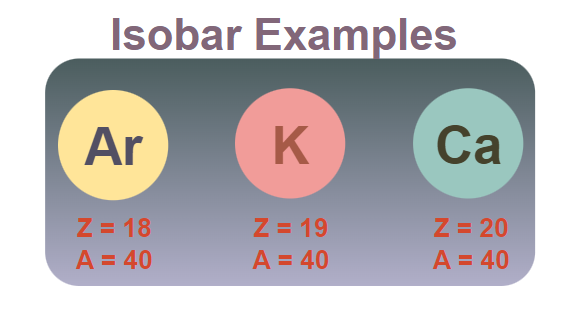# Isobars

Isobars are a group of elements that have the same mass number but different atomic numbers. In an isobar, we have different numbers of protons but the same number of nucleons, i.e. the sum of the number of protons and neutrons in the nucleus of isobars of the elements are the same. An example of isobar is carbon-14 and nitrogen-14 as they both have 14 nucleons in their nucleus but different atomic numbers, the atomic number of carbon is 6 and the atomic number of nitrogen is 7. The isobar has somewhat the same physical properties but different chemical properties.

In this article, we will learn about isobars, their examples, their differences with isotopes and others in detail.

## Isobars Definition

Isobars are a group of elements from the periodic table that have different atomic numbers but their mass number are the same. We can say that in isobars the number of protons in their nucleus is different but the sum of the number of protons and neutrons is the same. For example, Argon (18Ar40), Potassium  (19K40), and Calcium (20Ca40) are isobars as they all have 40 as their mass number but their atomic number are different. This happens because they have different atomic numbers but the sum of protons and neutrons in their nucleus is different. The table added below shows the following condition,

The image added below shows the group of Isobar of Argon, Potsaaium, and Calcium.## Isobars Examples

There are various examples in the periodic table that are isobars, i.e. they have the same mass number but different atomic numbers. Various examples of the isobars are discussed below,

11Na24 and12Mg24

Sodium(24) and Magnesium(24) are the isobars of each other and we can represent their condition as,

13Al27 and 14Si27

Aluminium(27) and Silicon(27) are the isobars of each other and we can represent their condition as,

16S34 and 17Cl34

Sulphur(34) and Chlorine(34) are the isobars of each other and we can represent their condition as,

26Fe58 and 27Ni58

Iron(58) and Nickel(58) are the isobars of each other and we can represent their condition as,

## Difference between Isotopes and Isobars

Isotopes and isobars are the elements that are widely used in chemistry and the basic difference between them is discussed below in the table.

## FAQs on Isobar

### Q1: What are Isobars?

Isobars in chemistry are defined as groups of elements that have different atomic numbers but similar mass numbers. The number of protons in the isobar is different but the sum of proton and neutron is the same.

### Q2: What are Examples of Isobars?

Various examples of the isobars in chemistry are,

• C-14 and N-14

Here, both carbon and nitrogen have the same mass number but different atomic numbers. The atomic number of the carbon is 6 and the atomic number of the nitrogen is 7. Some other examples of isobars are,

• Ar-40, K-40, and Ca-40
• S-34 and Cl-34

### Q3: How do Isobars differ from Isotopes?

Isotopes and isobars are widely used concepts in chemistry and they differ from each other in many ways such that, the isotopes are the different variants of the same chemical element, but isobars are the different chemical elements with the same mass number.

### Q4: What are the Uses of Isobars in Chemistry?

There are various uses of the isobar that includes,

• They are used in radiometric dating, in which the decay of radioactive isobars helps determines the age of organic materials.
• Isobars are used in nuclear physics to study various atomic nuclei, etc.

### Q5: How can we Identify Isobars?

We can identify the isobars using various techniques that include mass spectrometry, etc. Also, we can identify the isobars by studying the nuclear properties of their nucleus. In general, if two elements have the same mass number then they are isobar of each other.